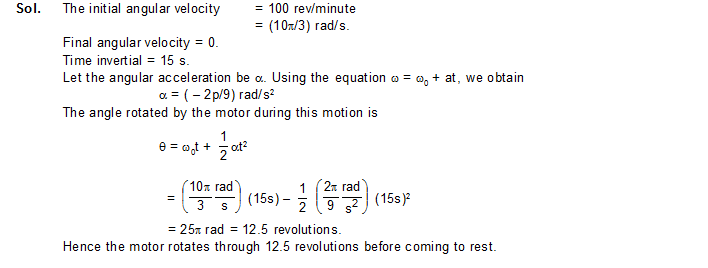# `SOLVED EXAMPLES`

1.    The motor of an engine is rotating about its axis with an angular velocity of 100 rev/minute. It comes to rest in 15 s, after being switched off. Assuming constant angular deceleration, calculate the number of revolutions made by it before coming to rest.2.  Starting from rest, a fan takes five seconds to attain the maximum speed of 400 rpm (revolution per minute). Assuming constant acceleration, find the time taken by the fan in attaining half the maximum speed.3.  A bucket is being lowered down into a well through a rope passing over a fixed pulley of radius 10 cm. Assume that the rope does not slip on the pulley. Find the angular velocity and angular acceleration of the pulley at an instant when the bucket is going down at a speed of 20 cm/s and has an acceleration of 4.0 m/s2.4.  Consider a pulley fixed at its centre of mass by a clamp. A light rope is wound over it and the free end is tied to a block. The tension in the rope is T. (a) Write the forces acting on the pulley. How are they related? (b) locate the axis of rotation. (c) Find the torque of the forces about the axis of rotation.Sol.(a)    The forces on the pulley are (figure)
(i)    attraction by the earth, Mg vertically downward,
(ii)    tension T by the rope, along the rope,
(iii)    contact force N by the support at the centre N = T + Mg (centre of mass of the pulley         is at rest, so Newton’s 1st law applies).
(b)    The axis of rotation is the line through the centre of the pulley and perpendicular to         the plane of the pulley.
(c)    Let us take the positive direction of the axis towards the reader.
The forces Mg passes through the centre of mass and it intersects the axis of rotation. Hence the torque of Mg about the axis is zero (Case- II). Similarly, the torque of the contact force N is also zero.
The tension T is along the tangent of the rim in the vertically downward direction. The tension and the axis of rotation are perpendicular but never intersect. Case III applies. Join the point where the rope leaves the rim to the centre. This line is the common perpendicular to the tension and the axis. Hence the torque is T.r (positive, since it will try to rotate the pulley anticlockwise).

5.    A wheel of radius 10 cm can rotate freely about its centre as shown in figure. A string is wrapped over its rim and is pulled by a force of 5.0 N. It is found that the torque produces an angular acceleration 2.0 rad/s in the wheel. Calculate the moment of the wheel.

[Image_Link]http://studymaterialkota.com/uploaded_files/userfiles/